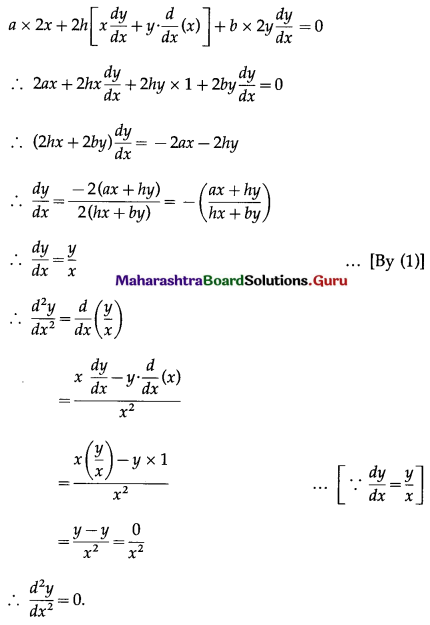Balbharati Maharashtra State Board 12th Commerce Maths Solution Book Pdf Chapter 3 Differentiation Miscellaneous Exercise 3 Questions and Answers.

## Maharashtra State Board 12th Commerce Maths Solutions Chapter 3 Differentiation Miscellaneous Exercise 3

(I) Choose the correct alternative:

Question 1.
If y = (5x3 – 4x2 – 8x)9, then $$\frac{d y}{d x}$$ = ___________
(a) 9(5x3 – 4x2 – 8x)8 (15x2 – 8x – 8)
(b) 9(5x3 – 4x2 – 8x)9 (15x2 – 8x – 8)
(c) 9(5x3 – 4x2 – 8x)8 (5x2 – 8x – 8)
(d) 9(5x3 – 4x2 – 8x)9 (5x2 – 8x – 8)
(a) 9(5x3 – 4x2 – 8x)8 (15x2 – 8x – 8)

Question 2.
If y = $$\sqrt{x+\frac{1}{x}}$$, then $$\frac{d y}{d x}$$ = ?
(a) $$\frac{x^{2}-1}{2 x^{2} \sqrt{x^{2}+1}}$$
(b) $$\frac{1-x^{2}}{2 x^{2} \sqrt{x^{2}+1}}$$
(c) $$\frac{x^{2}-1}{2 x \sqrt{x} \sqrt{x^{2}+1}}$$
(d) $$\frac{1-x^{2}}{2 x \sqrt{x} \sqrt{x^{2}+1}}$$
(c) $$\frac{x^{2}-1}{2 x \sqrt{x} \sqrt{x^{2}+1}}$$
Hint: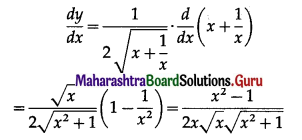Question 3.
If y = $$e^{\log x}$$ then $$\frac{d y}{d x}$$ = ?
(a) $$\frac{e^{\log x}}{x}$$
(b) $$\frac{1}{x}$$
(c) 0
(d) $$\frac{1}{2}$$
(a) $$\frac{e^{\log x}}{x}$$Question 4.
If y = 2x2 + 22 + a2, then $$\frac{d y}{d x}$$ = ?
(a) x
(b) 4x
(c) 2x
(d) -2x
(b) 4x

Question 5.
If y = 5x . x5, then $$\frac{d y}{d x}$$ = ?
(a) 5x . x4(5 + log 5)
(b) 5x . x5(5 + log 5)
(c) 5x . x4(5 + x log 5)
(d) 5x . x5(5 + x log 5)
(c) 5x . x4(5 + x log 5)

Question 6.
If y = $$\log \left(\frac{e^{x}}{x^{2}}\right)$$ then $$\frac{d y}{d x}$$ = ?
(a) $$\frac{2-x}{x}$$
(b) $$\frac{x-2}{x}$$
(c) $$\frac{e-x}{ex}$$
(d) $$\frac{x-e}{ex}$$
(b) $$\frac{x-2}{x}$$
Hint: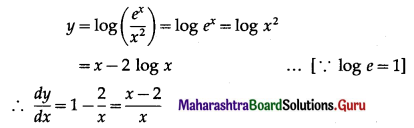Question 7.
If ax2 + 2hxy + by2 = 0, then $$\frac{d y}{d x}$$ = ?
(a) $$\frac{(a x+h y)}{(h x+b y)}$$
(b) $$\frac{-(a x+h y)}{(h x+b y)}$$
(c) $$\frac{(a x-h y)}{(h x+b y)}$$
(d) $$\frac{(2 a x+h y)}{(h x+3 b y)}$$
(b) $$\frac{-(a x+h y)}{(h x+b y)}$$

Question 8.
If x4 . y5 = (x + y)(m+1) and $$\frac{d y}{d x}=\frac{y}{x}$$ then m = ?
(a) 8
(b) 4
(c) 5
(d) 20
(a) 8
Hint:
If xp . yq = (x + y)p+q, then $$\frac{d y}{d x}=\frac{y}{x}$$
∴ m + 1 = 4 + 5 = 9
∴ m = 8.Question 9.
If x = $$\frac{e^{t}+e^{-t}}{2}$$, y = $$\frac{e^{t}-e^{-t}}{2}$$ then $$\frac{d y}{d x}$$ = ?
(a) $$\frac{-y}{x}$$
(b) $$\frac{y}{x}$$
(c) $$\frac{-x}{y}$$
(d) $$\frac{x}{y}$$
(d) $$\frac{x}{y}$$
Hint: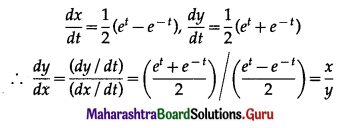Question 10.
If x = 2at2, y = 4at, then $$\frac{d y}{d x}$$ = ?
(a) $$-\frac{1}{2 a t^{2}}$$
(b) $$\frac{1}{2 a t^{3}}$$
(c) $$\frac{1}{t}$$
(d) $$\frac{1}{4 a t^{3}}$$
(c) $$\frac{1}{t}$$

(II) Fill in the blanks:

Question 1.
If 3x2y + 3xy2 = 0 then $$\frac{d y}{d x}$$ = …………
-1
Hint:
3x2y + 3xy2 = 0
∴ 3xy(x + y) = 0
∴ x + y = 0
∴ y = -x
∴ $$\frac{d y}{d x}$$ = -1

Question 2.
If xm . yn = (x+y)(m+n) then $$\frac{d y}{d x}=\frac{\ldots \ldots}{x}$$
y

Question 3.
If 0 = log(xy) + a then $$\frac{d y}{d x}=\frac{-y}{\ldots . .}$$
xQuestion 4.
If x = t log t and y = tt then $$\frac{d y}{d x}$$ = …………
y
Hint:
x = t log t = log tt = log y
∴ 1 = $$\frac{1}{y} \cdot \frac{d y}{d x}$$
∴ $$\frac{d y}{d x}$$ = y

Question 5.
If y = x . log x then $$\frac{d^{2} y}{d x^{2}}$$ = …………..
$$\frac{1}{x}$$

Question 6.
If y = [log(x)]2 then $$\frac{d^{2} y}{d x^{2}}$$ = …………..
$$\frac{2(1-\log x)}{x^{2}}$$
Hint: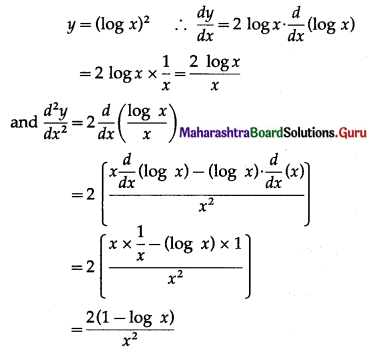Question 7.
If x = y + $$\frac{1}{y}$$ then $$\frac{d y}{d x}$$ = …………
$$\frac{y^{2}}{y^{2}-1}$$
Hint: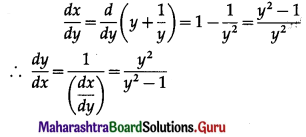Question 8.
If y = eax, then x.$$\frac{d y}{d x}$$ = …………
axy

Question 9.
If x = t . log t, y = tt then $$\frac{d y}{d x}$$ = …………
yQuestion 10.
If y = $$\left(x+\sqrt{x^{2}-1}\right)^{m}$$ then $$\sqrt{\left(x^{2}-1\right)} \frac{d y}{d x}$$ = ………
my
Hint: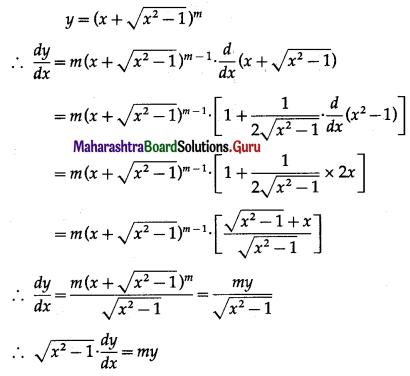(III) State whether each of the following is True or False:

Question 1.
If f’ is the derivative of f, then the derivative of the inverse of f is the inverse of f’.
False

Question 2.
The derivative of loga x, where a is constant is $$\frac{1}{x \cdot \log a}$$.
True

Question 3.
The derivative of f(x) = ax, where a is constant is x . ax-1
False

Question 4.
The derivative of a polynomial is polynomial.
TrueQuestion 5.
$$\frac{d}{d x}\left(10^{x}\right)=x \cdot 10^{x-1}$$
False

Question 6.
If y = log x, then $$\frac{d y}{d x}=\frac{1}{x}$$.
True

Question 7.
If y = e2, then $$\frac{d y}{d x}$$ = 2e.
False

Question 8.
The derivative of ax is ax. log a.
True

Question 9.
The derivative of xm . yn = (x + y)(m+n) is $$\frac{x}{y}$$
False

(IV) Solve the following:

Question 1.
If y = (6x3 – 3x2 – 9x)10, find $$\frac{d y}{d x}$$
Solution:
Given y = (6x3 – 3x2 – 9x)10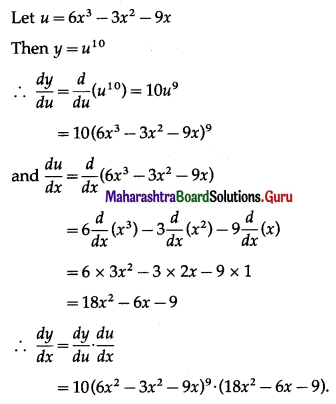Question 2.
If y = $$\sqrt{\left(3 x^{2}+8 x+5\right)^{4}}$$, find $$\frac{d y}{d x}$$.
Solution: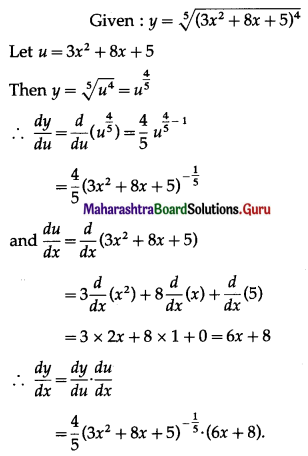Question 3.
If y = [log(log(log x))]2, find $$\frac{d y}{d x}$$.
Solution: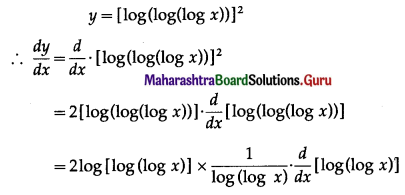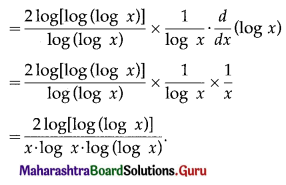Question 4.
Find the rate of change of demand (x) of a commodity with respect to its price (y) if y = 25 + 30x – x2.
Solution: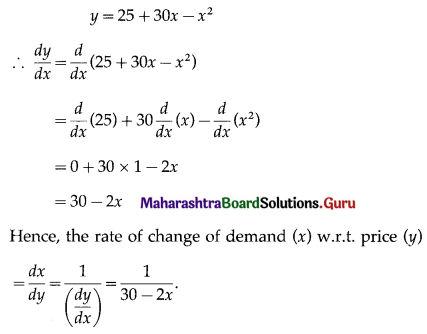Question 5.
Find the rate of change of demand (x) of a commodity with respect to its price (y) if y = $$\frac{5 x+7}{2 x-13}$$
Solution: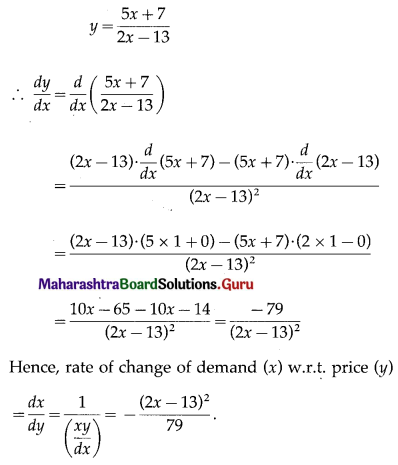Question 6.
Find $$\frac{d y}{d x}$$ if y = xx.
Solution:
y = xx
∴ log y = log xx = x log x
Differentiating both sides w.r.t. x, we get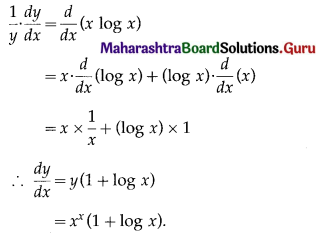Question 7.
Find $$\frac{d y}{d x}$$ if y = $$2^{x^{x}}$$.
Solution: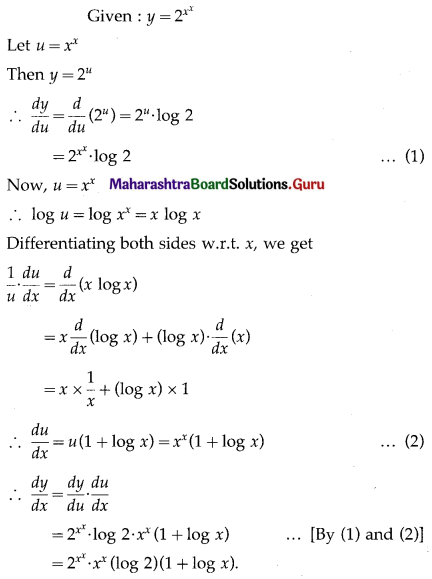Question 8.
Find $$\frac{d y}{d x}$$, if y = $$\sqrt{\frac{(3 x-4)^{3}}{(x+1)^{4}(x+2)}}$$
Solution: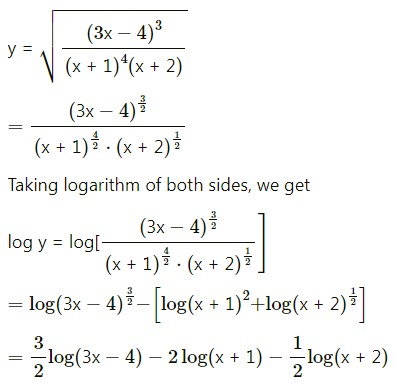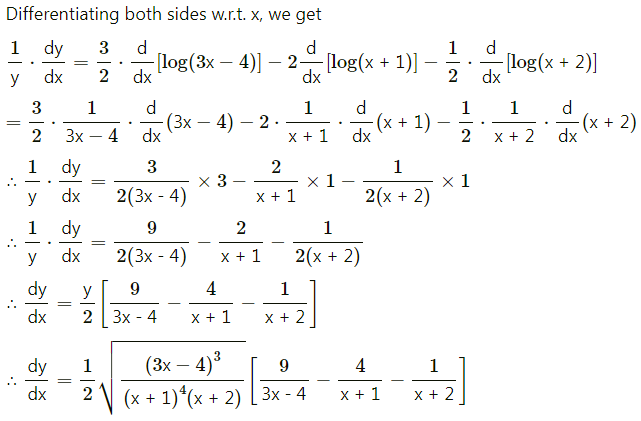Question 9.
Find $$\frac{d y}{d x}$$ if y = xx + (7x – 1)x
Solution: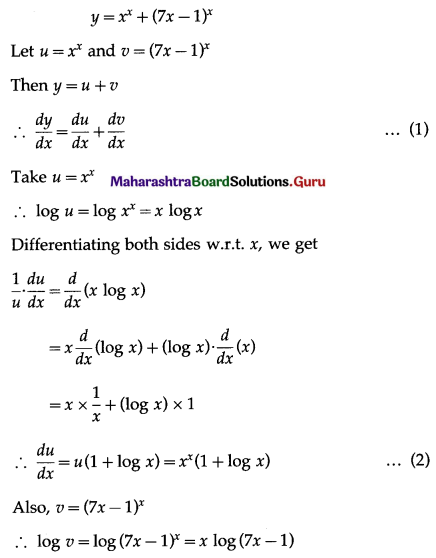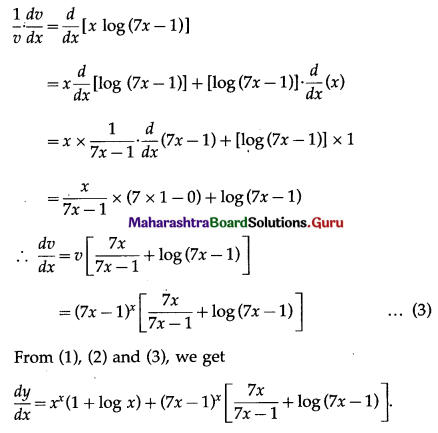Question 10.
If y = x3 + 3xy2 + 3x2y, find $$\frac{d y}{d x}$$.
Solution:
y = x3 + 3xy2 + 3x2y
Differentiating both sides w.r.t. x, we get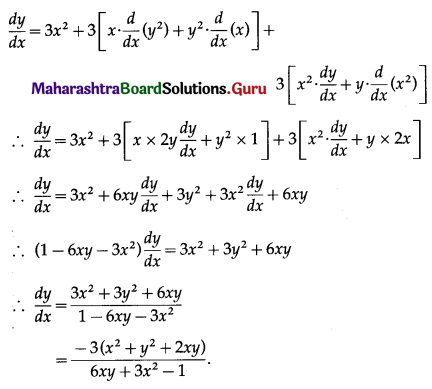Question 11.
If x3 + y2 + xy = 7, find $$\frac{d y}{d x}$$.
Solution:
x3 + y2 + xy = 7
Differentiating both sides w.r.t. x, we get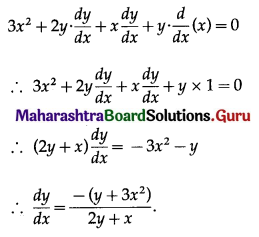Question 12.
If x3y3 = x2 – y2, find $$\frac{d y}{d x}$$.
Solution:
x3y3 = x2 – y2
Differentiating both sides w.r.t. x, we get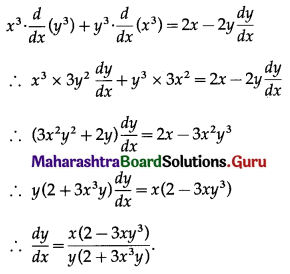Question 13.
If x7 . y9 = (x + y)16, then show that $$\frac{d y}{d x}=\frac{y}{x}$$.
Solution: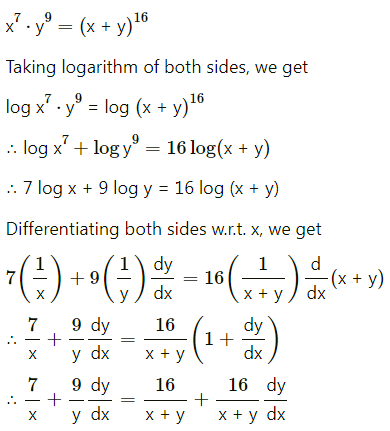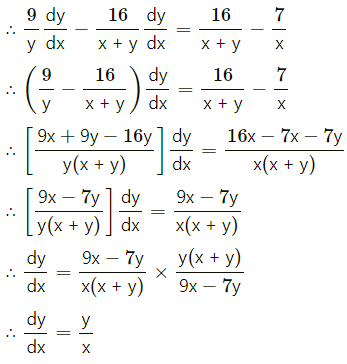Question 14.
If xa . yb = (x + y)a+b, then show that $$\frac{d y}{d x}=\frac{y}{x}$$.
Solution: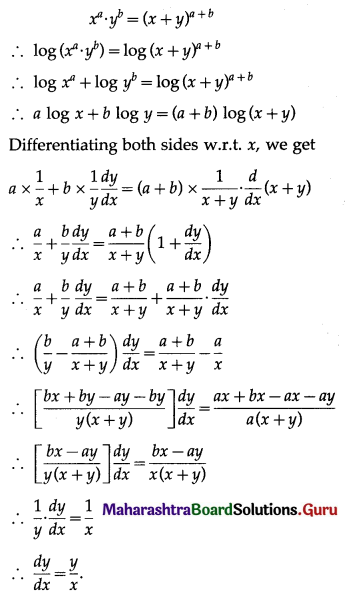Question 15.
Find $$\frac{d y}{d x}$$ if x = 5t2, y = 10t.
Solution:
x = 5t2, y = 10t
Differentiating x and y w.r.t. t, we get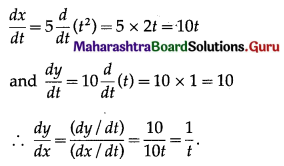Question 16.
Find $$\frac{d y}{d x}$$ if x = e3t, y = $$e^{\sqrt{t}}$$.
Solution:
x = e3t, y = $$e^{\sqrt{t}}$$
Differentiating x and y w.r.t. t, we get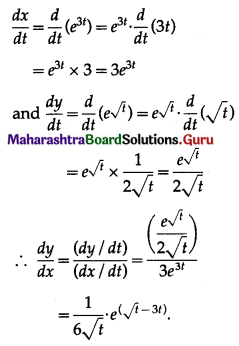Question 17.
Differentiate log(1 + x2) with respect to ax.
Solution:
Let u = log(1 + x2) and v = ax
Then we want to find $$\frac{d u}{d v}$$
Differentiating u and v w.r.t. x, we get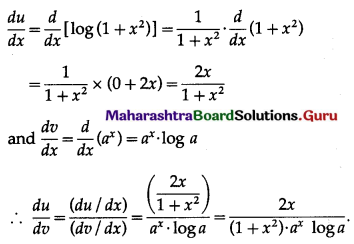Question 18.
Differentiate e(4x+5) with resepct to 104x.
Solution:
Let u = e(4x+5) and v = 104x
Then we want to find $$\frac{d u}{d v}$$
Differentiating u and v w.r.t. x, we get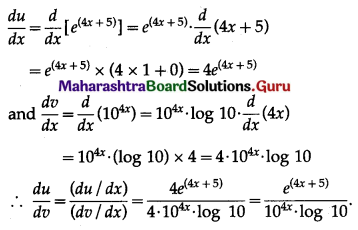Question 19.
Find $$\frac{d^{2} y}{d x^{2}}$$, if y = log x.
Solution:
y = log x
Differentiating w.r.t. x, we get
$$\frac{d y}{d x}=\frac{d}{d x}(\log x)=\frac{1}{x}$$
Differentiating again w.r.t. x, we get
$$\frac{d^{2} y}{d x^{2}}=\frac{d}{d x}\left(\frac{1}{x}\right)=-\frac{1}{x^{2}}$$

Question 20.
Find $$\frac{d^{2} y}{d x^{2}}$$, if y = 2at, x = at2.
Solution:
x = at2, y = 2at
Differentiating x and y w.r.t. t, we get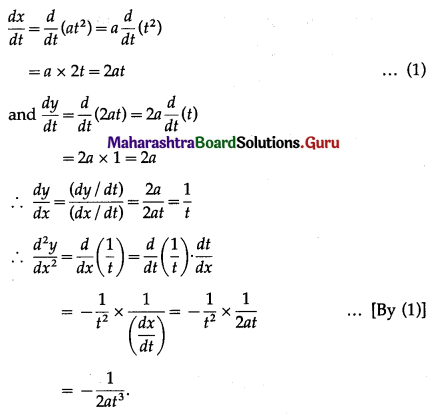Question 21.
Find $$\frac{d^{2} y}{d x^{2}}$$, if y = x2 . ex
Solution:
y = x2 . ex
Differentiating w.r.t. x, we get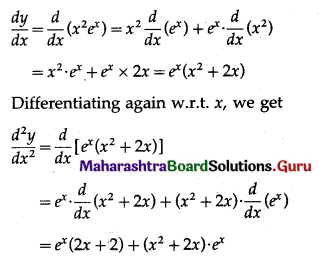= ex (2x + 2 + x2 + 2x)
= ex (x2 + 4x + 2).Question 22.
If x2 + 6xy + y2 = 10, then show that $$\frac{d^{2} y}{d x^{2}}=\frac{80}{(3 x+y)^{3}}$$.
Solution:
x2 + 6xy + y2 = 10 ……..(1)
Differentiating both sides w.r.t. a, we get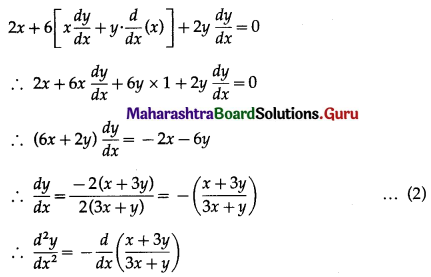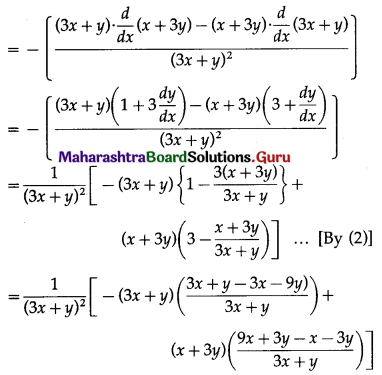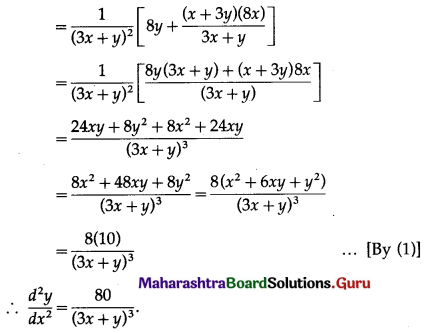Question 23.
If ax2 + 2hxy + by2 = 0, then show that $$\frac{d^{2} y}{d x^{2}}$$ = 0.
Solution:
ax2 + 2hxy + by2 = 0 ……..(1)
∴ ax2 + hxy + hxy + by2 = 0
∴ x(ax + hy) + y(hx + by) = 0
∴ x(ax + hy) = -y(hx + by)
∴ $$\frac{a x+h y}{h x+b y}=-\frac{y}{x}$$ …….(2)
Differentiating (1) w.r.t. x, we get